# NCERT Solutions for Class 8 Maths Chapter 3 Understanding quadrilaterals Exercise 3.1

In this page we have NCERT Solutions for Class 8 Maths Chapter 3 Understanding quadrilaterals for EXERCISE 3.1 .This exercise has questions about Angle Sum Property of Polygons, Convex and concave polygons,Regular and irregular polygons. Hope you like them and do not forget to like , social share and comment at the end of the page.

## NCERT Solutions for Class 8 Maths Chapter 3 Exercise 3.1

Question 1
Given here are some figures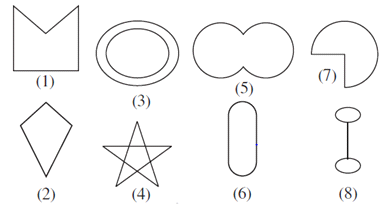Classify each of them on the basis of the following.
(a) Simple curve
(b) Simple closed curve
(c) Polygon
(d) Convex polygon
(e) Concave polygon
Answer(a) 1, 2, 5, 6, 7
(b) 1, 2, 5, 6, 7
(c) 1, 2
(d) 2
(e) 1

Question 2
How many diagonals does each of the following have?
(b) A regular hexagon
(c) A triangle
(a) There are 2 diagonals in a convex quadrilateral.
(b) There are 9 diagonals in a regular hexagon.
(c) A triangle does not have any diagonal in it.

Question 3
What is the sum of the measures of the angels of a convex quadrilateral? Will this property hold if the quadrilateral is not convex? (Make a non-convex quadrilateral and try!)
The sum of the measures of the angles of a convex quadrilateral is 360° as a convex quadrilateral is made of two triangles.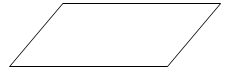In above convex quadrilateral, it made of two triangles. Therefore, the sum of all the interior angles of this quadrilateral will be same as the sum of all the interior angles of these two triangles i.e., 180º + 180º = 360º
This property also holds true for a quadrilateral which is not convex. This is because any quadrilateral can be divided into two triangles.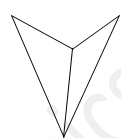Here again, above concave quadrilateral is made of two triangles. Therefore, sum of all the interior angles of this quadrilateral will also be 180º + 180º = 360º

Question 4
Examine the table. (Each figure is divided into triangles and the sum of the angles deduced from that.)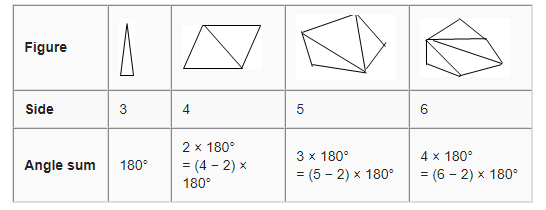What can you say about the angle sum of a convex polygon with number of sides?
(a) 7
(b) 8
(c) 10
(d) n
From the table, it can be observed that the angle sum of a convex polygon ofnsides is (n−2) × 180º.
So the angle sum of the convex polygons having number of sides as above will be as follows.
(a) (7 − 2) × 180º = 900°
(b) (8 − 2) × 180º = 1080°
(c) (10 − 2) × 180º = 1440°
(d) (n− 2) × 180°

Question 5
What is a regular polygon?
State the name of a regular polygon of
(i) 3 sides
(ii) 4 sides
(iii) 6 sides
A polygon with equal sides and equal angles is called a regular polygon.
(i) Equilateral Triangle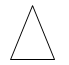(ii) Square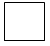(iii) Regular Hexagon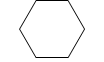Question 6
Find the angle measurexin the following figures.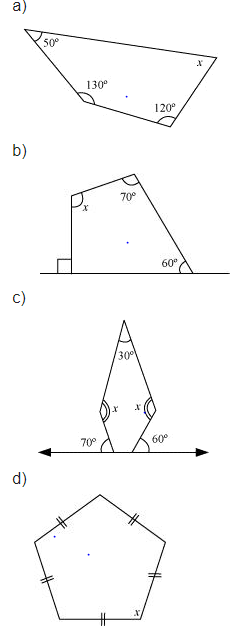(a)
Sum of the measures of all interior angles of a quadrilateral is 360°. Therefore, in the given quadrilateral,
50° + 130° + 120° +x= 360°
300° +x= 360°
x= 60°
(b)
Let the other unknown angle be p,then 90 and p forms a linear pair
90º +p= 180º (Linear pair)
p= 180º − 90º = 90º
Sum of the measures of all interior angles of a quadrilateral is 360º. Therefore, in the given quadrilateral,
60° + 70° +x+ 90° = 360°
220° +x= 360°
x= 140°
(c)
Let the other unknown angle be p and q in the pentagon,
then
70 +p= 180° (Linear pair)
p= 110°
60° +q= 180° (Linear pair)
q= 120°
Sum of the measures of all interior angles of a pentagon is
=540º.
Therefore, in the given pentagon,
120° + 110° + 30° +x + x= 540°
260° + 2x= 540°
2x= 280°
x= 140°
(d)
Sum of the measures of all interior angles of a pentagon is 540º.
5x= 540°
x= 108°

Question 7
(a)find $x + y + z$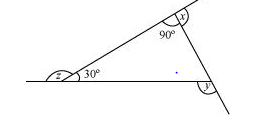(b)find $x + y + z+w$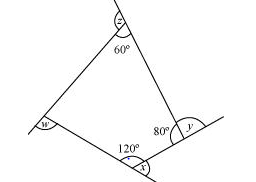(a) x+ 90° = 180° (Linear pair)
x= 90°
z+ 30° = 180° (Linear pair)
z= 150°
y= 90° + 30° (Exterior angle theorem)
y= 120°
x + y + z= 90° + 120° + 150° = 360°
(b)
Sum of the measures of all interior angles of a quadrilateral is 360º. Therefore, in the given quadrilateral,
a+ 60° + 80° + 120° = 360°
a+ 260° = 360°
a= 100°
x+ 120° = 180° (Linear pair)
x= 60°
y+ 80° = 180° (Linear pair)
y= 100°
z+ 60° = 180° (Linear pair)
z= 120°
w+ 100° = 180° (Linear pair)
w= 80°
Sum of the measures of all interior angles =x + y + z + w
= 60° + 100° + 120° + 80°
= 360°

## Summary

1. NCERT solutions for class 8 maths chapter 3 Understanding quadrilaterals Exercise 3.1 has been prepared by Expert with utmost care. If you find any mistake.Please do provide feedback on mail. You can download the solutions as PDF in the below Link also
2. This chapter 3 has total 4 Exercise 3.1 ,3.2,3.3 and 3.4. This is the first exercise in the chapter.You can explore previous exercise of this chapter by clicking the link below